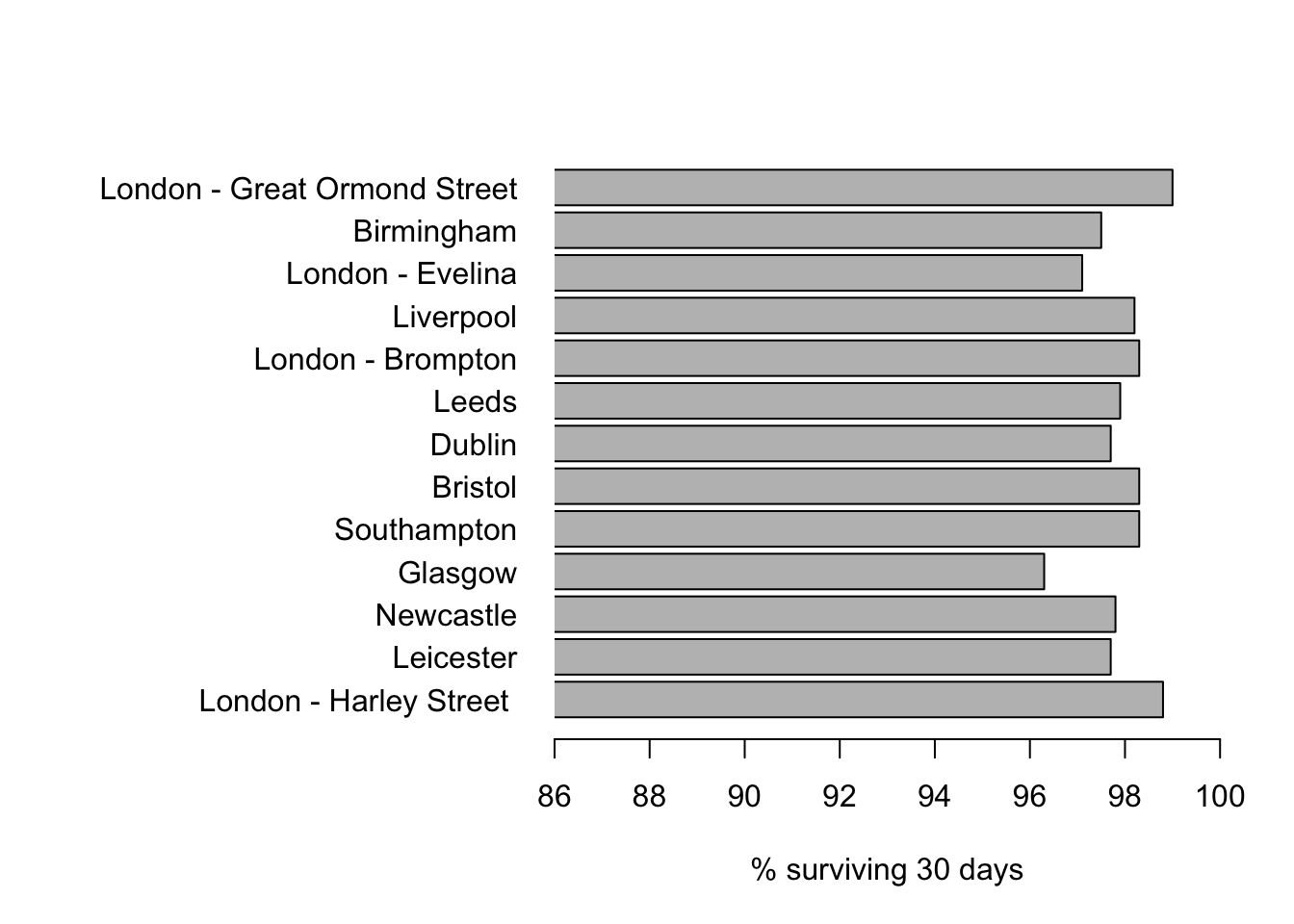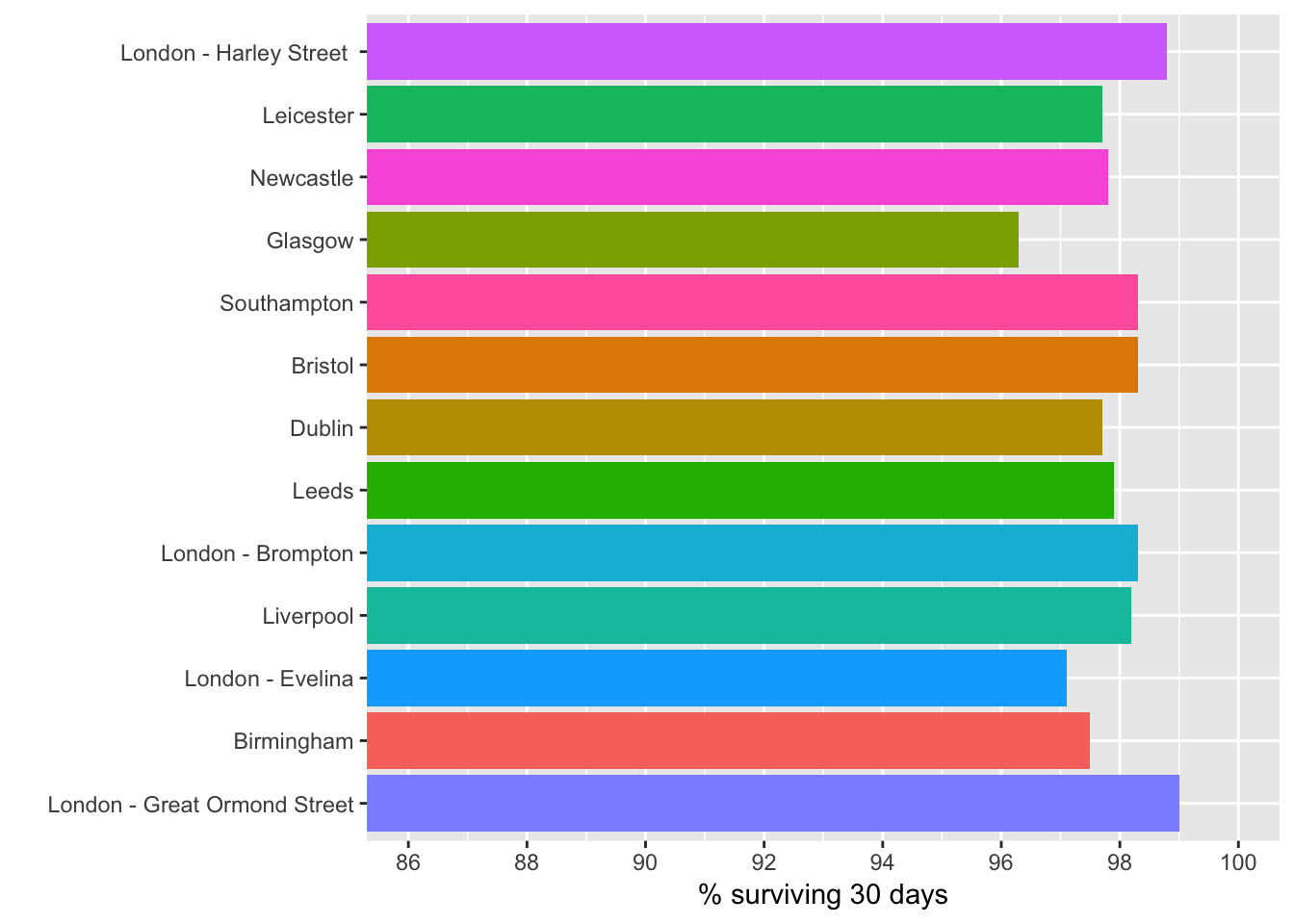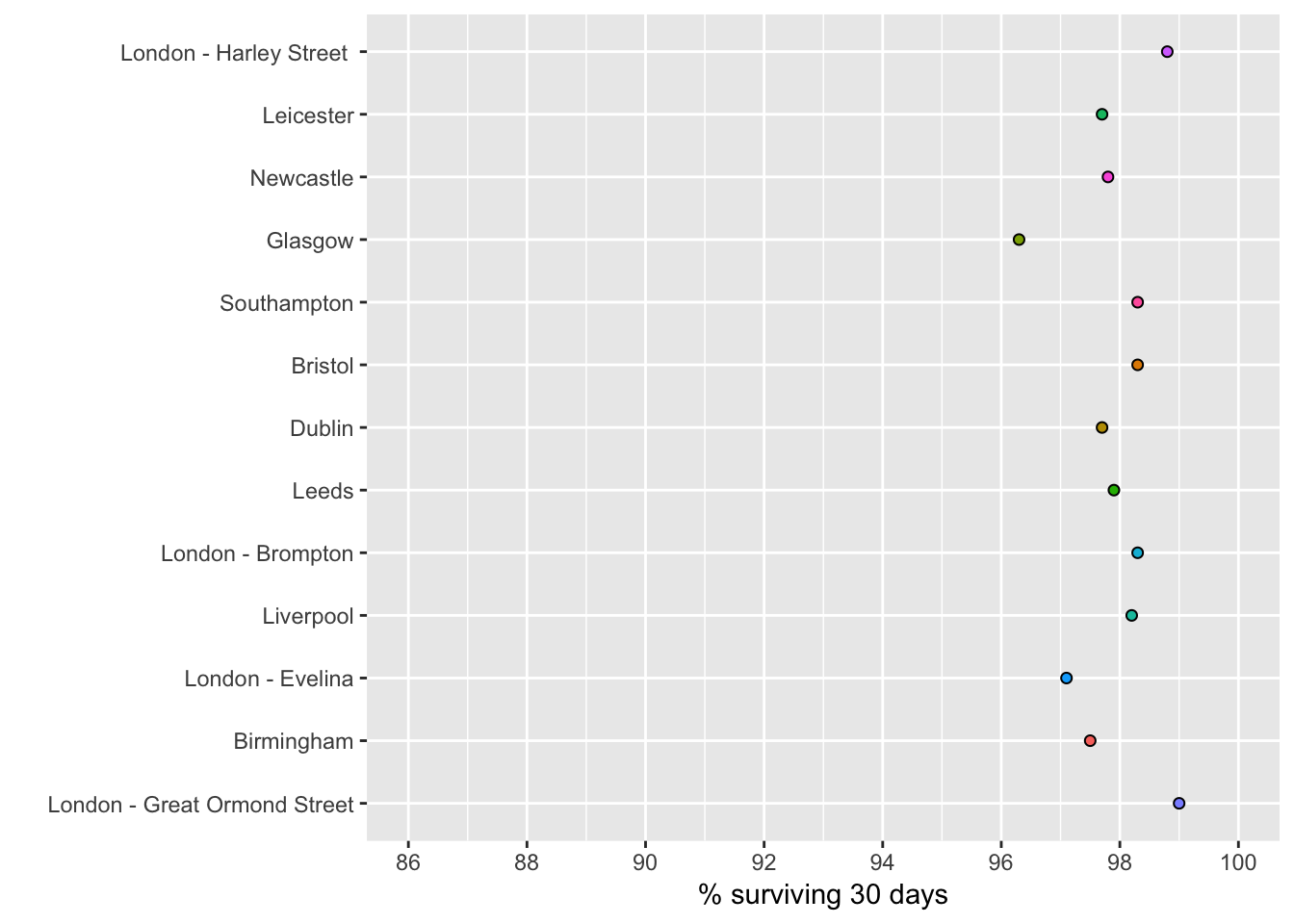Figure 1.1: Survival rates following child heart surgery in thirteen hospitals from 2012-2015

Data are shown in Table 1.1 (page 23) and are contained in 01-1-child-heart-survival-x.csv. The data were originally presented in the NCHDA 2012-15 report, but are best seen on childrensheartsurgery.info.

library(ggplot2)

attach(ThirtyDaySurv)
nhosp=length(Hospital)

First in R base graphics

par(mar=c(5,15,4,2))
barplot(ThirtyDaySurvival,names.arg=Hospital,horiz=T,xlim=c(86,100), xpd=F,las=1, xlab="% surviving 30 days")Now in ggplot2

p <- ggplot(ThirtyDaySurv, aes(x=reorder(Hospital,nhosp:1), y= ThirtyDaySurvival, fill=Hospital)) # constructs initial plot object, , starting with top row
p <- p + geom_bar(stat = "identity") # assigns bar chart-type
p <- p + coord_flip(ylim = c(86,100)) # flips to horizontal bars and limits y-axis
p <- p + scale_y_continuous(breaks=seq(86, 100, 2)) # assigns breaks every 2 percent
p <- p + scale_colour_brewer(palette = "Accent") # sets the colour palette
p <- p + theme(legend.position="none") # removes the legend
p <- p +  labs(x="", y="% surviving 30 days") # Adds y-axis label
p # draws the plotFigure 1.1 Bar-chart of 30-day survival rates for thirteen hospitals. The choice of the start of the horizontal axis, here 86%, can have a crucial effect on the impression given by the graphic. If the axis starts at 0%, all the hospitals will look indistinguishable, whereas if we started at 95% the differences would look misleadingly dramatic.

For other ways of displaying and explaining this data, and more recent results, see childrensheartsurgery.info, which uses a dot-plot. This may be more appropriate.

p <- ggplot(ThirtyDaySurv, aes(x=reorder(Hospital,nhosp:1), y= ThirtyDaySurvival, fill=Hospital)) # constructs initial plot object, , starting with top row
p <- p + geom_dotplot(binaxis="y",stackdir="center",dotsize=2) # assigns dots chart-type
p <- p + coord_flip(ylim = c(86,100)) # flips to horizontal bars and limits y-axis
p <- p + scale_y_continuous(breaks=seq(86, 100, 2)) # assigns breaks every 2 percent
p <- p + theme(legend.position="none") # removes the legend
p <- p +  labs(x="", y="% surviving 30 days") # Adds y-axis label
p # draws the plot
## stat_bindot() using bins = 30. Pick better value with binwidth.Figure 1.1 Bar-chart of 30-day survival rates for thirteen hospitals represented as a dot-plot, in which the non-zero axis is less important as, unlike a bar, it is not directly connected to the data-point.